# E-PolyLearning

 6. What is Nusselt number ?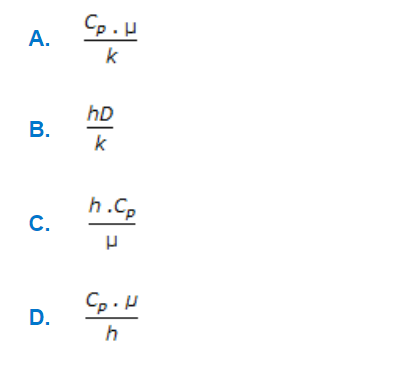a. A b. B c. C d. D

 7. The Dietus-Boelter equation for convective heat transfer given by following equation, cannot be used for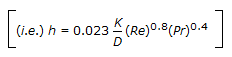a. low Reynold's number b. very low Grashoff number c. molten metals d. all (a), (b) and (c)
 8. The rate of heat transfer through a pipe wall is given by following equation. For cylinder of very thin wall, q can be approximated by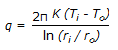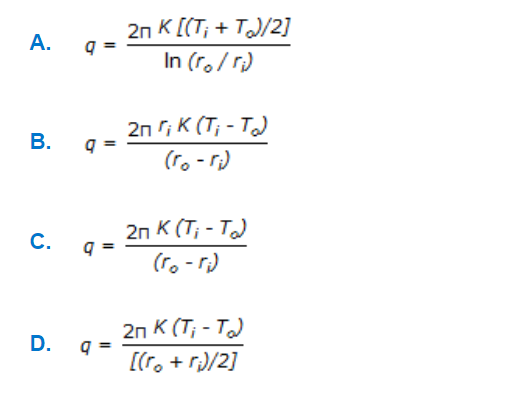a. A b. B c. C d. D
 9. Graetz number is given by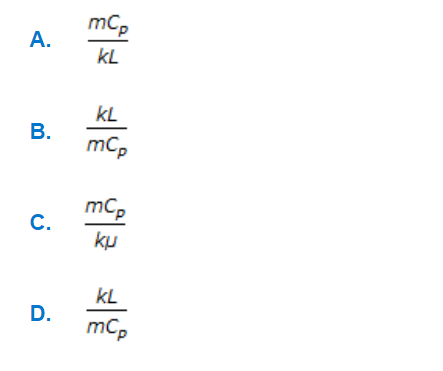a. A b. B c. C d. D
 10. Harmonic mean temperature difference is given by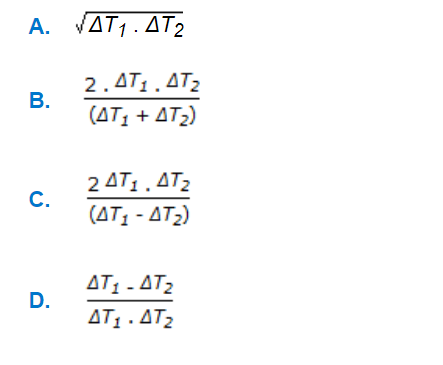a. A b. B c. C d. D